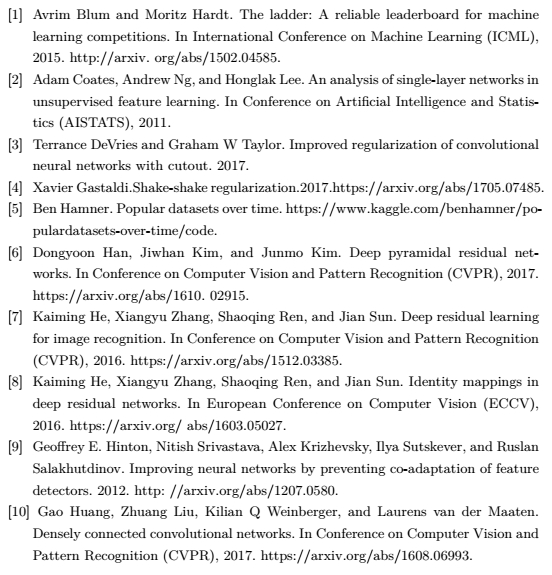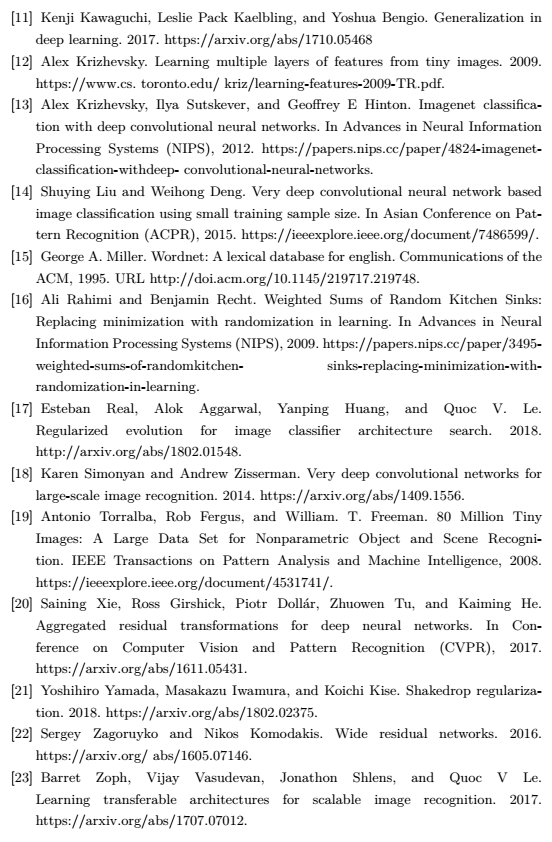**

# Do CIFAR-10 Classifiers Generalize to CIFAR-10?

**

## 1.1 一个CIFAR-10的重复性研究

1. 首先，我们需要建立一个新的测试集，将我们新测试集的子类分布与原始CIFAR-10数据集仔细匹配。
2. 收集了大约2000张新图像后，我们在新的测试集上评估了30个图像分类模型的性能。结果显示了两个总体现象。一方面，从原始测试集到我们的新测试集的准确度都有明显下降。例如，VGG和ResNet架构在我们新的测试集上从93％的精确度下降到85％左右。另一方面，我们发现现有测试集的性能对新测试集的性能具有高度的预测性。即使是对CIFAR-10的小幅增量改进，也会有明显的泛化误差。
3. 由于原始精度和新精度之间的差异，第三步调查了解释这种差距的多种假设。一个自然而然的猜想是，重新调整标准超参数可以恢复一些观察到的差距，但我们发现只有一小部分效果提高了约0.6％。虽然这个和进一步的实验可以解释一些准确度损失，但仍然存在重大差距。

## 2 正式实施

$\begin{array}{}\text{(9)}& L{}_{\mathit{D}\mathit{\left(}\stackrel{\mathit{\to }}{\mathit{f}}\mathit{\right)}}={\mathbb{E}}_{\left(\left(x,y\right)\sim D\right)}\left[\mathbb{I}\left[\stackrel{\to }{f}\left(x\right)\ne y\right]\right].\end{array}$

$\begin{array}{}\text{(10)}& {\mathit{L}}_{{D}_{test}}\left(\stackrel{\to }{f}\right)=\frac{1}{|{D}_{test}|}\sum _{\left(x,y\right)\in {D}_{test}}^{}\mathbb{I}|\stackrel{\to }{f}\left(x\right)\ne y|.\end{array}$

$\begin{array}{}\text{(11)}& {\mathit{L}}_{D}\left(\stackrel{\to }{f}\right)\right)={L}_{{D}_{test}\stackrel{\to }{\left(}f\right)}\approx {L}_{{D}_{test}^{{}^{\prime }}\stackrel{\to }{\left(}f\right)}.\end{array}$

## 3 数据集创建方式

• 目前，CIFAR-10是机器学习中使用最广泛的数据集之一，并可作为许多计算机视觉方法的测试集。CIFAR-10是NIPS-2017（MNIST之后）中第二个最常用的数据集。
• CIFAR-10的数据集创建过程透明且有据可查。更重要的是，CIFAR-10是从具有更多细粒度标签的更大的Tiny Images存储库中抽取。这使我们可以进行一项实验，使我们的新测试集中的各种形式的分布变化程度最小化。
• CIFAR-10提出了一个足够困难的问题，因此数据集仍然是积极研究的主题（参见）。此外，还有大量的分类模型可以获得显着不同的准确性成绩。由于这些模型的代码是在各种开源代码库中发布的，因此它们可以被视为独立于我们的新测试集。

## 3.1 背景

Tiny Image 该数据集包含8千万个分辨率为$32\ast 32$$32*32$的RGB彩色图像。图像由大约75,000个关键字组成，这些关键字与WordNet数据库中的非抽象名词相对应。每个关键字都被输入到多个互联网搜索引擎中，每个关键词收集大约1,000到2,500个图像。需要注意的是，Tiny Images是一个相当嘈杂的数据集。根据某个关键字提交的许多图像并不清楚（或根本不对应）相应的关键字。
CIFAR-10 CIFAR-10数据集的目标是创建一个干净标记的Tiny Images子集。为此，研究人员组建了一个由10个类组成的数据集，每个类有6,000个图像。这些类是飞机，汽车，鸟，猫，鹿，狗，青蛙，马，船和卡车。标准的训练/测试划分是均衡的，包含50,000个训练图像集和10,000个测试图像集。
CIFAR-10的创建过程已有详细记录。首先，研究人员通过使用WordNet中的下位关系为每个类汇集了一组相关的关键字。由于直接使用来自Tiny Images的相应图像不会提供高质量的数据集，因此研究人员聘请学生注释人员标注了来自Tiny Images的图像。标签说明可以在的附录C中找到，并且包括一组特定的指导（例如，图像不应该包含相应类别的两个对象）。研究人员验证由注释者选择的图像的标签后，通过“${l}_{2}$$l_{2}$最近邻居搜索”从数据集中去除近似重复的数据。

## 3.2 构建新的测试集

• 虽然最初的CIFAR-10测试集包含10,000张图像，但对于相当小的置信区间，大小为2,000的测试集已经足够。具体而言，对于准确度90％的保守置信区间（Clopper-Pearson，置信水平95％）具有约1％的大小，其中$n=2,000$$n=2,000$（准确地说，[88：6％;91：3％]）。由于我们认为原始测试精度与新测试精度之间的潜在差异只有在测量精度大于1％时才有意义，因此我们认为新的测试集大小为2,000，足以满足我们的研究需求。
• 与非常罕见的关键字一样，我们的目标是避免意外地创建更困难的测试集。由于一些微小图像关键字只剩下有限的剩余图像，因此我们认为新数据集的使用较小目标尺寸会减少偏差以避免包含更多可疑难度的图像。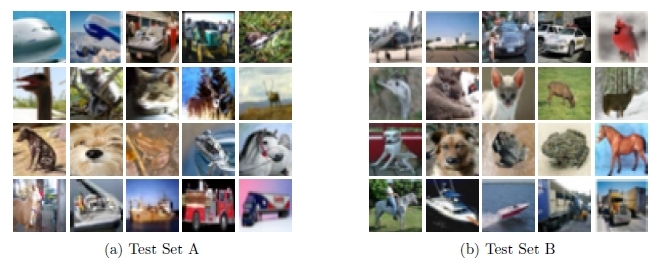## 4.2 性能的相对顺序几乎没有变化

$\begin{array}{}\text{(12)}& ac{c}_{new}=\left(1.62±0.04\right)\cdot ac{c}_{orig}-65.51\mathrm{%}±3.16\end{array}$

## 4.3 线性拟合的一个模型

$\begin{array}{}\text{(13)}& ac{c}_{orig}={p}_{1}\cdot {a}_{0}+{p}_{2}\cdot \left(1-k\left(1-{a}_{0}\right)\right).\end{array}$

$\begin{array}{}\text{(14)}& ac{c}_{orig}=\beta \cdot {a}_{0}+\gamma .\end{array}$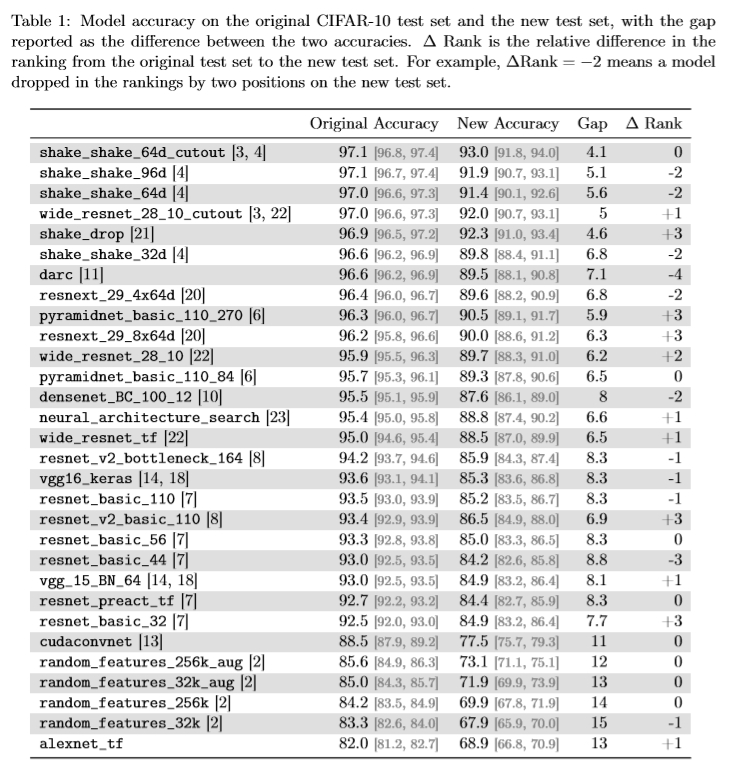$\begin{array}{}\text{(15)}& ac{c}_{new}={q}_{1}\cdot {a}_{0}+{q}_{2}\cdot \left(1-k\left(1-{a}_{0}\right)\right)={\beta }^{{}^{\prime }}\cdot {a}_{0}+{\gamma }^{{}^{\prime }}.\end{array}$

$\begin{array}{}\text{(16)}& ac{c}_{new}=\frac{{\beta }^{{}^{\prime }}}{\beta }\left(\beta {a}_{0}+\gamma \right)-\frac{{\beta }^{{}^{\prime }}}{\beta }\gamma +{\gamma }^{{}^{\prime }}=\frac{{\beta }^{{}^{\prime }}}{\beta }ac{c}_{orig}.\end{array}$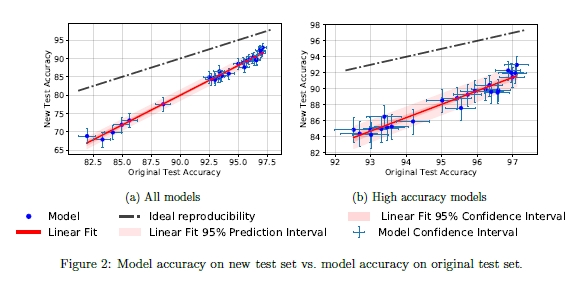## 5.3 超参数调整

• 学习率在集合$0.0125,0.025,0.05,0.1,0.2,0.4,0.8$${0.0125,0.025,0.05,0.1,0.2,0.4,0.8}$
• 下降率在集合$0.5,0.75,1.125,1.75$${0.5,0.75,1.125,1.75}$
• 权值减少在集合$5e-5,1e-4,5e-4,1e-3,5e-3$${5e-5,1e-4,5e-4,1e-3,5e-3}$

## 5.6 交叉验证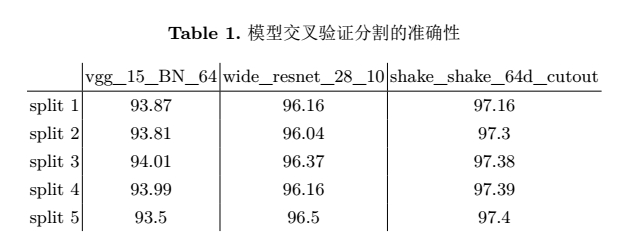## 6 讨论

• 训练集过度训练。量化过度拟合的一种方法是培训准确性和测试准确性之间的差异。请注意，我们实验中的深度神经网络通常达到100％的训练精度。所以这种过度拟合的概念已经出现在现有的数据集上。
• 测试集过度拟合。过度拟合的另一个概念是测试准确性和基础数据分布的准确性之间的差距。通过将模型设计选择适配于测试集，这里值得关注的是，我们隐含地将模型拟合范围扩大到测试集。然后，测试准确性将失去其有效性，从而很难准确衡量真正没有预先学习的数据集，其这样的性能也不会很好。

## 引用